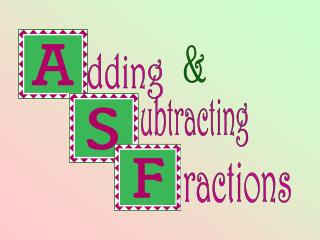DownloadDownload Presentationractions

# ractions

Télécharger la présentation## ractions

- - - - - - - - - - - - - - - - - - - - - - - - - - - E N D - - - - - - - - - - - - - - - - - - - - - - - - - - -
##### Presentation Transcript

1. & dding ubtracting ractions

2. Adding & Subtracting FractionsTeacher Notes: • Use this presentation to teach the steps for adding and subtracting fractions with unlike denominators. • It is assumed that students have a thorough understanding of the process for making equivalent fractions. • CLICK HERE to view a demonstration with opportunities for students to practice making equivalent fractions.

3. We need a common denominator to add these fractions. +

4. We need a common denominator to add these fractions. + Count by 2's 2, 4, 6, 8, 10, 12, 14, 16, 18, 20 Count by 7's 7, 14, 21, 28, 35…

5. Count by 2's 2, 4, 6, 8, 10, 12, 14, 16, 18, 20 Count by 7's 7, 14, 21, 28, 35… The first number IN COMMON that appears on both lists becomes the common denominator

6. x 7 7 Make equivalent fractions. = X 7 x 2 6 Add the numerators = + 7 + 6 = 13 x 2 13

7. We need a common denominator to add these fractions. + Count by 7's 7, 14, 21, 28, 35, 42, 49, 56, 63 Count by 5's 5, 10, 15, 20, 25, 30, 35, 40, 45

8. x 5 15 Make equivalent fractions. = X 5 x 7 7 + Add the numerators = x 7 15 + 7 = 22 22

9. We need a common denominator to add these fractions. + Count by 7's 7, 14, 21, 28, 35, 42, 49, 56, 63 Count by 8's 8, 16, 24, 32, 40, 48, 56, 64, 72

10. 32 x 8 Make equivalent fractions. = x 8 21 Add the numerators x 7 + 32 + 21 = 53 = x 7 53

11. We need a common denominator to add these fractions. + Count by 3's 3, 6, 9, 12, 15, 18, 21, 24, 27 Count by 5's 5, 10, 15, 20, 25, 30, 35, 40, 45

12. x 3 9 Add the numerators = X 3 9 + 10 = 19 x 5 10 + = x 5 19

13. We need a common denominator to add these fractions. + Count by 3's 3, 6, 9, 12, 15, 18, 21, 24, 27, 30, 33 Count by 11's 11, 22, 33, 44, 55, 66, 77…

14. 15 x 3 Make equivalent fractions. = X 3 x 11 Add the numerators 22 + = 15 + 22 = 37 x 11 37

15. We need a common denominator to add these fractions. + Count by 3's 3, 6, 9, 12, 15, 18, 21, 24, 27 Count by 6's 6, 12, 18, 24,…

16. 4 x 2 = X 2 Make equivalent fractions. 1 x 1 + Add the numerators = x 1 4 + 1 = 5 5

17. We need a common denominator to add these fractions. + Count by 11's 11, 22, 33, 44, 55, 66, 77… 6, 12, 18, 24, 30, 36, 42, 48, 54, 60, 66, 72

18. x 6 18 Make equivalent fractions. = X 6 x 11 11 + Add the numerators. = 18 + 11 = 29 x 11 29

19. We need a common denominator to add these fractions. +

20. We need a common denominator to add these fractions. + Count by 5's 5, 10, 15, 20, 25, 30, 35, 40, 45 Count by 9's 9, 18, 27, 36, 45, 54, 63, 72, 81

21. Count by 5's 5, 10, 15, 20, 25, 30, 35, 40, 45 Count by 9's 9, 18, 27, 36, 45, 54, 63, 72, 81 The first number IN COMMON that appears on both lists becomes the common denominator

22. x 9 36 Make equivalent fractions. Make equivalent fractions. = x9 x 5 35 Add the numerators. Add the numerators. + = 36 + 35 = 71 36 + 35 = 71 x 5 71

23. We need a common denominator to add these fractions. + Count by 12's 12, 24, 36, 48, 60, 72, 84, 96 Count by 8's 8, 16, 24, 32, 40, 48, 56, 64, 72, 79, 80, 88, 96

24. 10 x 2 Make equivalent fractions. = x 2 9 x 3 Add the numerators. + = 10 + 9 = 19 x 3 19

25. We need a common denominator to subtract a fraction from another. - Count by 5's 5, 10, 15, 20, 25, 30, 35, 40, 45, 50, 55 Count by 11's 11, 22, 33, 44, 55, 66, 77…

26. 15 x 5 Make equivalent fractions. = x 5 11 x 11 Subtract. = 15 - 11 = 4 - x 11 4

27. We need a common denominator to subtract one fraction from another. - Count by 2's 2, 4, 6, 8, 10, 12, 14, 16, 18, 20 … Count by 10's 10, 20, 30…

28. 5 x 5 Make equivalent fractions. = x 5 3 x 1 Subtract. - = 5 – 3 = 2 x 1 2

29. Try These A D E B F C

30. Answers On Next Slide • Each click on the next slide reveals an answer. • Check your papers. • If you discover an incorrect answer, be able to explain your mistake.

31. 17 27 19 20 10 9 41 28 26 21 13 12 Try These A D B E C F